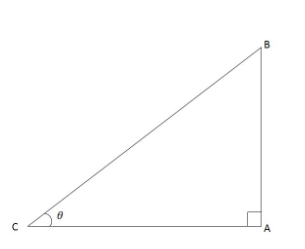QuestionAnswers

# The length of shadow of a tower is $\sqrt 3$ times that of its height. Find the angle of elevation of the sun.A.45°B.30°C.60°D.90°

Hint: Draw the figure of situation state in the question then use the proper trigonometric formula. The angle of elevation of an object as seen by the observer is defined as the angle between the horizontal and the line from the object to the observer’s eye. The line in which the observer's eye is there is known as the line of sight.Let ABC is a right angle triangle.
∠ ACB =$\theta$
Let the length of AB = height of tower = x.
So, shadow length = Length of BC = $\sqrt {3x}$.
In ∆ ACB,
$\tan \theta = \dfrac{{Perpendicular}}{{Base}}$
$\tan \theta = \dfrac{{AB}}{{BC}}$
$\tan \theta = \dfrac{x}{{\sqrt {3x} }}$
$\tan \theta = \dfrac{1}{{\sqrt 3 }}$
Tan θ = tan30° $\left( {\tan 30^\circ = \dfrac{1}{{\sqrt 3 }}} \right)$
$\theta$= 30°
So, the angle of elevation of the sun is 30°. So, from the above option only the B option is correct.

Note: We can also find the angle using trigonometric formula Cot $\theta$
i.e. $Cot \theta= \dfrac{{Base}}{{Perpendicular}}$. The angle of elevation is the angle between the horizontal and a line from your position to the sun.